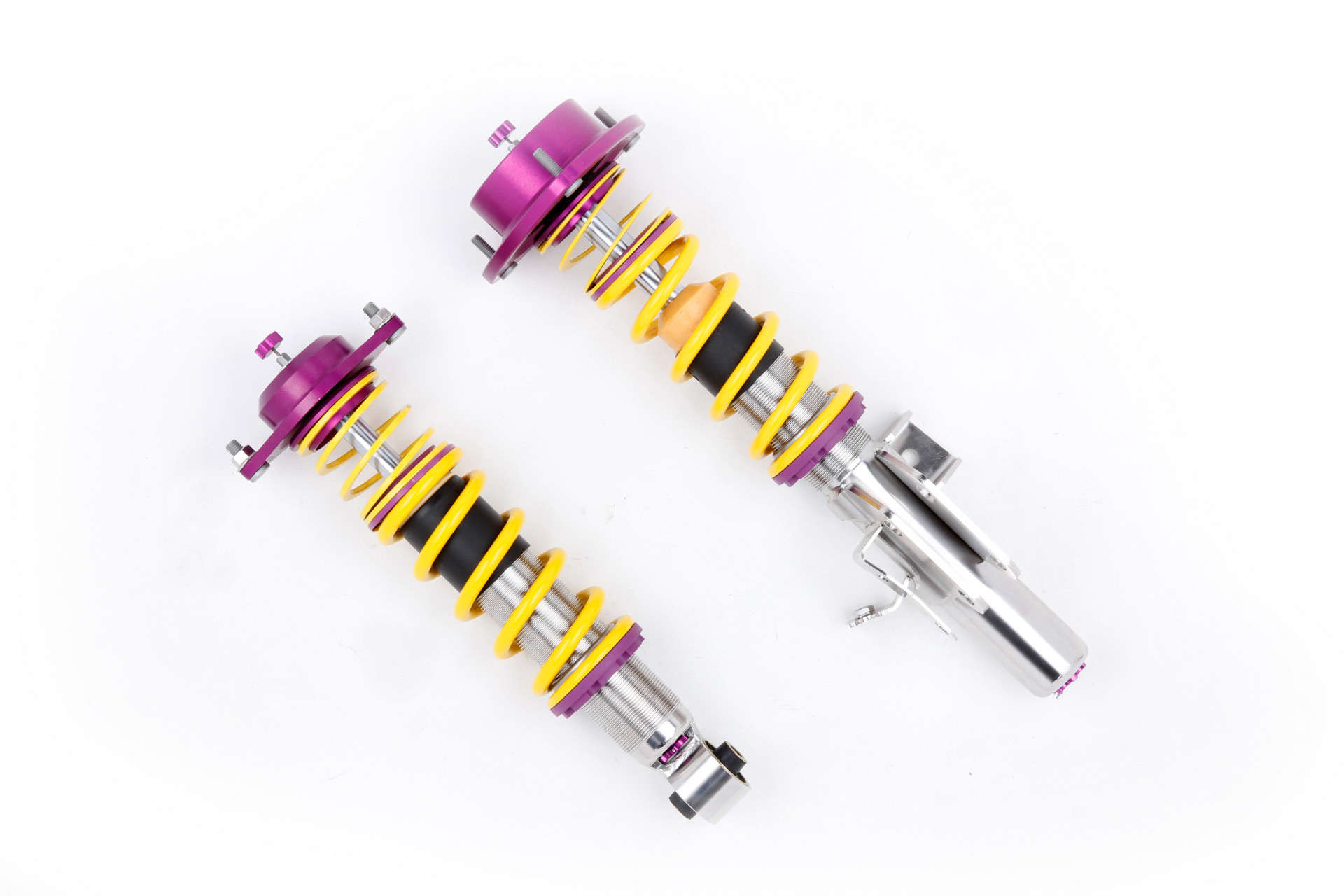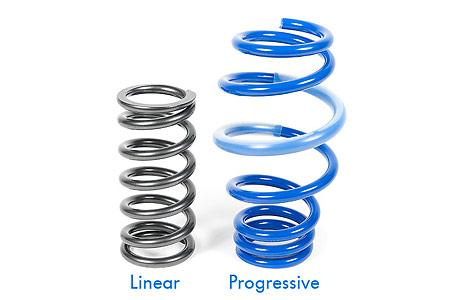# Linear vs. Progressive Springs Explained## What is spring rate?

Spring rate is the amount of weight required to compress a spring one inch.  The lower the spring rate the softer the spring, the softer the spring the smoother the ride.  If one applies a 200 pound load to a spring and it deflects an inch in length, the spring has a 200 pound spring rate.

## What are linear springs?

Linear springs have equal distance between each coil throughout the entire length of the spring.  With linear springs, the spring rate is constant and does not change by the load acting on the spring.  If one applies a 100 lb load to a spring with a rate of 100 lbs, the spring will depress one inch.  If we then apply an additional 100 lb load (200 lbs in total) the spring will depress another inch.  The result is still a 100 lb spring rating even when 200 lbs are applied since the spring will still depress 1 inch per every 100 lbs.  This is a linear ratio.

Linear springs react the same way under every condition, it's a simple ratio of weight applied to distance moved.  Because of this simple equation, linear springs are ideal for racing applications as one can easily predict handling around a track. Linear springs provide great driver feedback to the handling characteristics of the car.

Linear springs are very stiff, although ideal for handling on the track, they provide a rough ride for street use since they do not handle the smaller bumps well.

## What are progressive springs?

Progressive springs have unequal distance between each coil throughout the length of the spring.  The spring rate will change progressively with the compression of the spring.  This allows a single spring to have different spring rates.  As an example, an initial 100 lbs may be required to move the spring 1 inch, making the spring rate 100 lbs.  For the spring to travel another inch it may require 175 lbs.  The spring load “progresses” in weight required for it to compress each subsequent inch.  This progression in spring rate is made possible by the varying distances between each coil.